# Quartic plane curve

A quartic plane curve is a plane algebraic curve of the fourth degree. It can be defined by a bivariate quartic equation:with at least one of A, B, C, D, E not equal to zero. This equation has 15 constants. However, it can be multiplied by any non-zero constant without changing the curve; thus by the choice of an appropriate constant of multiplication, any one of the coefficients can be set to 1, leaving only 14 constants. Therefore, the space of quartic curves can be identified with the real projective space. It also follows that there is exactly one quartic curve that passes through a set of 14 distinct points in general position, since a quartic has 14 degrees of freedom.

A quartic curve can have a maximum of:

One may also consider quartic curves over other fields (or even rings), for instance the complex numbers. In this way, one gets Riemann surfaces, which are one-dimensional objects over C, but are two-dimensional over R. An example is the Klein quartic. Additionally, one can look at curves in the projective plane, given by homogeneous polynomials.

## Examples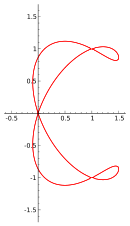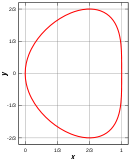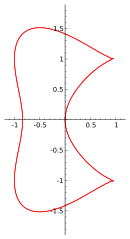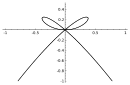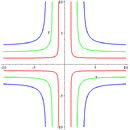Cruciform curve with parameters (b,a) being (1,1) in red; (2,2) in green; (3,3) in blue.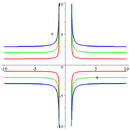Cruciform curve with parameters (b,a) being (1,1) in red; (2,1) in green; (3,1) in blue.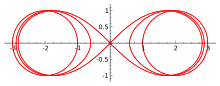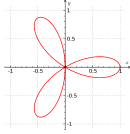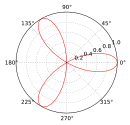Various combinations of coefficients in the above equation give rise to various important families of curves as listed below.

### Ampersand curve

The ampersand curve is a quartic plane curve given by the equation:It has genus zero, with three ordinary double points, all in the real plane. 

### Bean curve

The bean curve is a quartic plane curve with the equation:The bean curve has genus zero. It has one singularity at the origin, an ordinary triple point.  

### Bicuspid curve

The biscuspid is a quartic plane curve with the equationwhere a determines the size of the curve. The bicuspid has only the two nodes as singularities, and hence is a curve of genus one. 

### Bow curve

The bow curve is a quartic plane curve with the equation:The bow curve has a single triple point at x=0, y=0, and consequently is a rational curve, with genus zero. 

### Cruciform curve

The cruciform curve, or cross curve is a quartic plane curve given by the equationwhere a and b are two parameters determining the shape of the curve. The cruciform curve is related by a standard quadratic transformation, x ↦ 1/x, y ↦ 1/y to the ellipse a2x2 + b2y2 = 1, and is therefore a rational plane algebraic curve of genus zero. The cruciform curve has three double points in the real projective plane, at x=0 and y=0, x=0 and z=0, and y=0 and z=0. 

Because the curve is rational, it can be parametrized by rational functions. For instance, if a=1 and b=2, thenparametrizes the points on the curve outside of the exceptional cases where the denominator is zero.

### Spiric section

Main article: Spiric section

Spiric sections can be defined as bicircular quartic curves that are symmetric with respect to the xand y-axes. Spiric sections are included in the family of toric sections and include the family of hippopedes and the family of Cassini ovals. The name is from σπειρα meaning torus in ancient Greek.

### Three-leaved clover

The three-leaved clover is the quartic plane curveBy solving for y, the curve can be described by the following function:The parametric equation of three-leaved clover isIn polar coordinates (x = r cos φ, y = r sin φ) the equation isIt is a special case of rose curve with k = 3. This curve has a triple point at the origin (0, 0) and has three double tangents.

## References

1. Cundy, H. Martyn; Rollett, A. P. (1961) , Mathematical models (2nd ed.), Clarendon Press, Oxford, p. 72, ISBN 978-0-906212-20-2, MR 0124167
2. Gibson, C. G., Elementary Geometry of Algebraic Curves, an Undergraduate Introduction, Cambridge University Press, Cambridge, 2001, ISBN 978-0-521-64641-3. Pages 12 and 78.